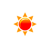# Meters to Feet Converter (m to ft)

 Example: 1 m = 3.28084 ft meter: m feet: ft
You may also interested in: feet to meters Converter

The online meter to ft Converter is used to convert the length from meters to feet.

#### The meter to feet Conversion Formula

You can use the following formula to convert from meter to feet :

X(feet) = y(m) ÷ 0.3048

Example: How to convert 100 meters to feet?

X(feet) = 100(m) ÷ 0.3048

Anwser: 328.08 ft

#### meters to feet conversion table

Meter (m) Feet (ft)
1 m 3.28084 ft
2 m 6.56168 ft
3 m 9.84252 ft
4 m 13.12336 ft
5 m 16.404199 ft
6 m 19.685039 ft
7 m 22.965879 ft
8 m 26.246719 ft
9 m 29.527559 ft
10 m 32.808399 ft
20 m 65.616798 ft
30 m 98.425197 ft
40 m 131.233596 ft
50 m 164.041995 ft
60 m 196.850394 ft
70 m 229.658793 ft
80 m 262.467192 ft
90 m 295.275591 ft
100 m 328.08399 ft
500 m 1640.419948 ft
1000 m 3280.839895 ft
Full meters to feet conversion table

#### Frequently asked questions to convert meters to feet

What is 2 meters in feet ?

What is 3 meters in feet ?

What is 4 meters in feet ?

What is 5 meters in feet ?

What is 6 meters in feet ?

What is 7 meters in feet ?

What is 8 meters in feet ?

What is 9 meters in feet ?

What is 10 meters in feet ?

What is 20 meters in feet ?

What is 30 meters in feet ?

#### Online Converter to convert meters to feet

To know how many feet in a meter, you can use the meter to feet converter to convert the meter to feet.

#### References

More references for Metre and Feet/Foot unit

#### Local Weather Status

Ashburn, US
11th November, 2019 Monday
Clear Sky17.78 - 21
Humidity: 34 %
Wind: 4.1 km/h